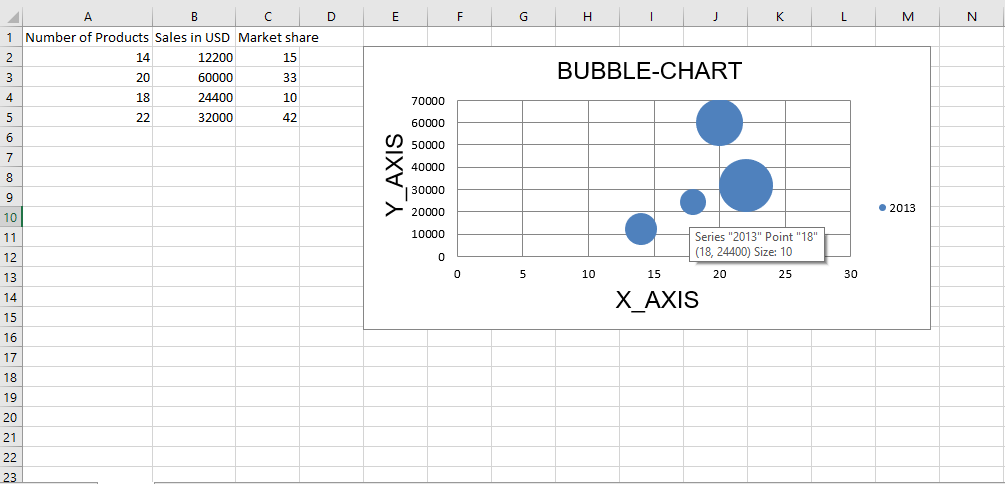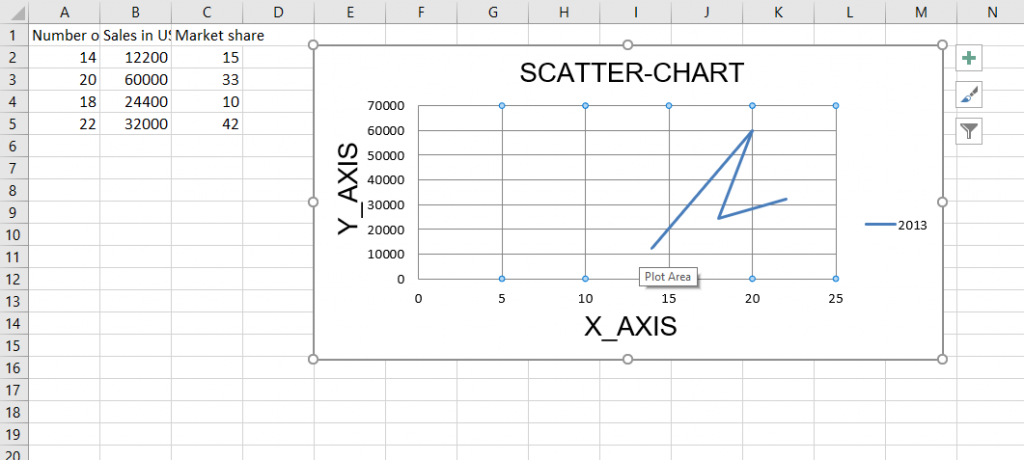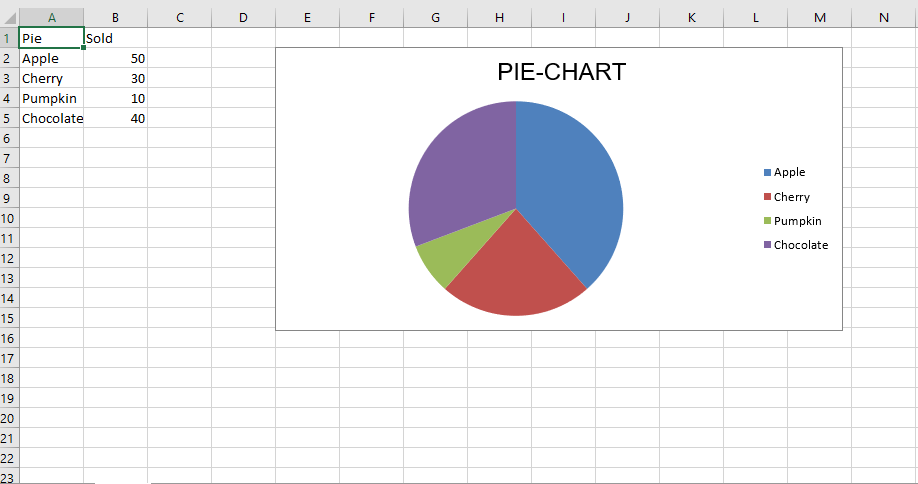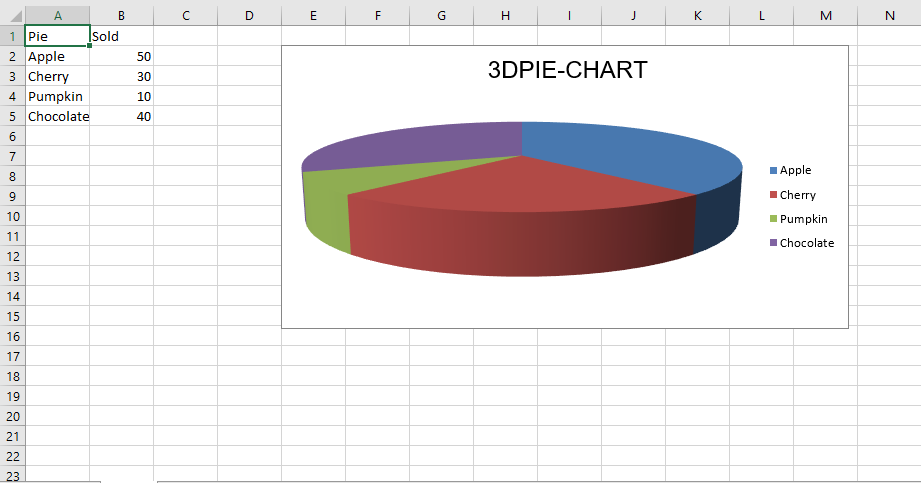# Python | Plotting charts in excel sheet using openpyxl module | Set – 2

Prerequisite: Python | Plotting charts in excel sheet using openpyxl module | Set – 1 Openpyxl is a Python library using which one can perform multiple operations on excel files like reading, writing, arithmetic operations and plotting graphs. Charts are composed of at least one series of one or more data points. Series themselves are comprised of references to cell ranges. Let’s see how to plot Scatter, Bubble, Pie, 3D Pie Chart on an excel sheet using openpyxl. For plotting the charts on an excel sheet, firstly, create chart object of specific chart class( i.e ScatterChart, PieChart etc.). After creating chart objects, insert data in it and lastly, add that chart object in the sheet object. Let’s see how to plot different charts using realtime data. Code #1 : Plot the Bubble Chart. Bubble charts are similar to scatter charts but use a third dimension to determine the size of the bubbles. Charts can include multiple series. For plotting the bubble chart on an excel sheet, use BubbleChart class from openpyxl.chart submodule.

## Python3

 `# import openpyxl module` `import` `openpyxl`   `# import BubbleChart, Reference, Series class` `# from openpyxl.chart sub_module` `from` `openpyxl.chart ``import` `BubbleChart, Reference, Series`   `# Call a Workbook() function of openpyxl ` `# to create a new blank Workbook object` `wb ``=` `openpyxl.Workbook()`   `# Get workbook active sheet ` `# from the active attribute.` `sheet ``=` `wb.active`   `rows ``=` `[` `    ``("Number of Products", "Sales ``in` `USD", "Market share"),` `    ``(``14``, ``12200``, ``15``),` `    ``(``20``, ``60000``, ``33``),` `    ``(``18``, ``24400``, ``10``),` `    ``(``22``, ``32000``, ``42``),` `]`   `# write content of each row in 1st, 2nd and 3rd` `# column of the active sheet respectively.` `for` `row ``in` `rows:` `    ``sheet.append(row)`   `# Create object of BubbleChart class` `chart ``=` `BubbleChart()`   `# create data for plotting` `xvalues ``=` `Reference(sheet, min_col ``=` `1``,` `                    ``min_row ``=` `2``, max_row ``=` `5``)` `                    `  `yvalues ``=` `Reference(sheet, min_col ``=` `2``,` `                    ``min_row ``=` `2``, max_row ``=` `5``)` `                    `  `size ``=` `Reference(sheet, min_col ``=` `3``,` `                ``min_row ``=` `2``, max_row ``=` `5``)`   `# create a 1st series of data` `series ``=` `Series(values ``=` `yvalues, xvalues ``=` `xvalues,` `                      ``zvalues ``=` `size, title ``=``"``2013``")`   `# add series data to the chart object` `chart.series.append(series)`   `# set the title of the chart` `chart.title ``=` `" BUBBLE``-``CHART "`   `# set the title of the x-axis` `chart.x_axis.title ``=` `" X_AXIS "`   `# set the title of the y-axis` `chart.y_axis.title ``=` `" Y_AXIS "`   `# add chart to the sheet` `# the top-left corner of a chart` `# is anchored to cell E2 .` `sheet.add_chart(chart, "E2")`   `# save the file` `wb.save("bubbleChart.xlsx")`

Output:Code #2 : Plot the Scatter Chart Scatter, or xy charts are similar to some line charts. For plotting the Scatter chart on an excel sheet, use ScatterChart class from openpyxl.chart submodule.

## Python3

 `# import openpyxl module` `import` `openpyxl`   `# import ScatterChart, Reference, Series` `# class from openpyxl.chart sub_module` `from` `openpyxl.chart ``import` `ScatterChart, Reference, Series`   `# Call a Workbook() function of openpyxl ` `# to create a new blank Workbook object` `wb ``=` `openpyxl.Workbook()`   `# Get workbook active sheet ` `# from the active attribute.` `sheet ``=` `wb.active`   `rows ``=` `[` `    ``("Number of Products", "Sales ``in` `USD", "Market share"),` `    ``(``14``, ``12200``, ``15``),` `    ``(``20``, ``60000``, ``33``),` `    ``(``18``, ``24400``, ``10``),` `    ``(``22``, ``32000``, ``42``),` `]`   `# write content of each row in 1st, 2nd and 3rd` `# column of the active sheet respectively .` `for` `row ``in` `rows:` `    ``sheet.append(row)`   `# Create object of ScatterChart class` `chart ``=` `ScatterChart()`   `# create data for plotting` `xvalues ``=` `Reference(sheet, min_col ``=` `1``,` `                    ``min_row ``=` `2``, max_row ``=` `5``)` `                    `  `yvalues ``=` `Reference(sheet, min_col ``=` `2``,` `                    ``min_row ``=` `2``, max_row ``=` `5``)` `                    `  `size ``=` `Reference(sheet, min_col ``=` `3``,` `                 ``min_row ``=` `2``, max_row ``=` `5``)`   `# create a 1st series of data` `series ``=` `Series(values ``=` `yvalues, xvalues ``=` `xvalues,` `                      ``zvalues ``=` `size, title ``=``"``2013``")`   `# add series data to the chart object` `chart.series.append(series)`   `# set the title of the chart` `chart.title ``=` `" SCATTER``-``CHART "`   `# set the title of the x-axis` `chart.x_axis.title ``=` `" X_AXIS "`   `# set the title of the y-axis` `chart.y_axis.title ``=` `" Y_AXIS "`   `# add chart to the sheet` `# the top-left corner of a chart` `# is anchored to cell E2 .` `sheet.add_chart(chart, "E2")`   `# save the file` `wb.save(" ScatterChart.xlsx")`

Output:Code #3 : Plot the Pie Chart Pie charts plot data as slices of a circle with each slice representing the percentage of the whole. Slices are plotted in a clockwise direction with 0° being at the top of the circle. Pie charts can only take a single series of data. For plotting the Pie chart on an excel sheet, use PieChart class from openpyxl.chart submodule.

## Python

 `# import openpyxl module` `import` `openpyxl`   `# import PieChart, Reference class` `# from openpyxl.chart sub_module` `from` `openpyxl.chart ``import` `PieChart, Reference`   `# Call a Workbook() function of openpyxl ` `# to create a new blank Workbook object` `wb ``=` `openpyxl.Workbook()`   `# Get workbook active sheet ` `# from the active attribute.` `sheet ``=` `wb.active`   `datas ``=` `[` `    ``[``'Pie'``, ``'Sold'``],` `    ``[``'Apple'``, ``50``],` `    ``[``'Cherry'``, ``30``],` `    ``[``'Pumpkin'``, ``10``],` `    ``[``'Chocolate'``, ``40``],` `]`   `# write content of each row in 1st, 2nd and 3rd` `# column of the active sheet respectively .` `for` `row ``in` `datas:` `    ``sheet.append(row)`   `# Create object of PieChart class` `chart ``=` `PieChart()`   `# create data for plotting` `labels ``=` `Reference(sheet, min_col ``=` `1``,` `                   ``min_row ``=` `2``, max_row ``=` `5``)` `                   `  `data ``=` `Reference(sheet, min_col ``=` `2``,` `                   ``min_row ``=` `1``, max_row ``=` `5``)`   `# adding data to the Pie chart object` `chart.add_data(data, titles_from_data ``=` `True``)`   `# set labels in the chart object` `chart.set_categories(labels)`   `# set the title of the chart` `chart.title ``=` `" PIE``-``CHART "`   `# add chart to the sheet` `# the top-left corner of a chart` `# is anchored to cell E2 .` `sheet.add_chart(chart, "E2")`   `# save the file` `wb.save(" PieChart.xlsx")`

Output:Code #4: Plot the Bar Chart For plotting the 3D pie chart on an excel sheet, use PieChart3D class from openpyxl.chart submodule.

## Python3

 `# import openpyxl module` `import` `openpyxl`   `# import PieChart3D, Reference class` `# from openpyxl.chart sub_module` `from` `openpyxl.chart ``import` `PieChart3D, Reference`   `# Call a Workbook() function of openpyxl ` `# to create a new blank Workbook object` `wb ``=` `openpyxl.Workbook()`   `# Get workbook active sheet ` `# from the active attribute.` `sheet ``=` `wb.active`   `datas ``=` `[` `    ``[``'Pie'``, ``'Sold'``],` `    ``[``'Apple'``, ``50``],` `    ``[``'Cherry'``, ``30``],` `    ``[``'Pumpkin'``, ``10``],` `    ``[``'Chocolate'``, ``40``],` `]`   `# write content of each row in 1st, 2nd and 3rd` `# column of the active sheet respectively .` `for` `row ``in` `datas:` `    ``sheet.append(row)`   `# Create object of PiChart3D class` `chart ``=` `PieChart3D()`   `# create data for plotting` `labels ``=` `Reference(sheet, min_col ``=` `1``,` `                   ``min_row ``=` `2``, max_row ``=` `5``)` `data ``=` `Reference(sheet, min_col ``=` `2``,` `                   ``min_row ``=` `1``, max_row ``=` `5``)`   `# adding data to the Pie chart object` `chart.add_data(data, titles_from_data ``=` `True``)`   `# set labels in the chart object` `chart.set_categories(labels)`   `# set the title of the chart` `chart.title ``=` `" ``3DPIE``-``CHART "`   `# add chart to the sheet` `# the top-left corner of a chart` `# is anchored to cell E2 .` `sheet.add_chart(chart, "E2")`   `# save the file` `wb.save(" ``3DPieChart``.xlsx")`

Output:Whether you're preparing for your first job interview or aiming to upskill in this ever-evolving tech landscape, GeeksforGeeks Courses are your key to success. We provide top-quality content at affordable prices, all geared towards accelerating your growth in a time-bound manner. Join the millions we've already empowered, and we're here to do the same for you. Don't miss out - check it out now!

Previous
Next